# 逆转形态：测试头肩形态

31 十二月 2018, 06:36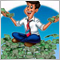0
3 875

### 1. 形态形成的理论观点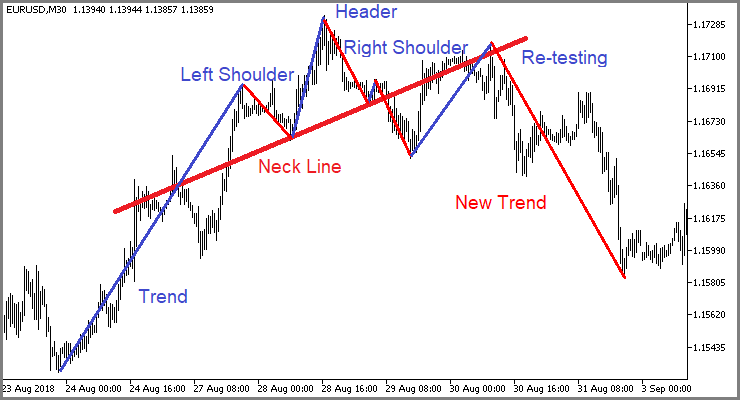### 2. 形态交易策略

#### 2.1. 案例 1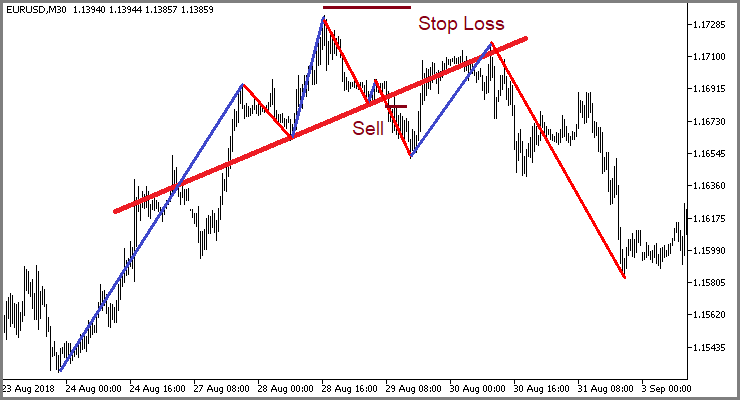#### 2.2. 案例 2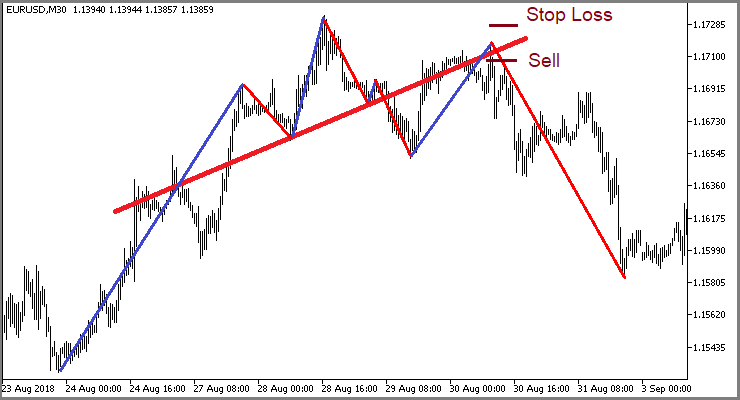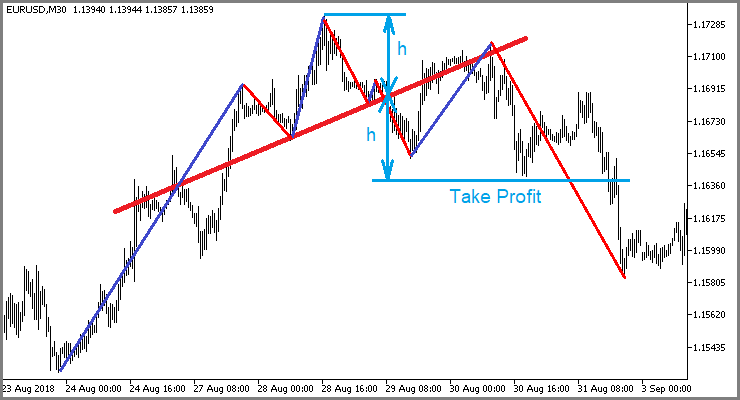### 3. 为进行策略测试而创建智能交易系统

```class CHS_Pattern : public CPattern
{
protected:
s_Extremum     s_StartRShoulder;       //右侧肩部起始点

public:
CHS_Pattern();
~CHS_Pattern();
//--- 初始化类
virtual bool      Create(CTrends *trends, double min_correction, double max_correction);
//--- 形态和入场点搜索方法
virtual bool      Search(datetime start_time);
virtual bool      CheckSignal(int &signal, double &sl, double &tp1, double &tp2);
//---
s_Extremum        StartRShoulder(void)    const {  return s_StartRShoulder;   }
};
```

```bool CHS_Pattern::Create(CTrends *trends,double min_correction,double max_correction)
{
if(!CPattern::Create(trends,min_correction,max_correction))
return false;
//---
s_StartRShoulder.Clear();
//---
return true;
}
```

```bool CHS_Pattern::Search(datetime start_time)
{
if(CheckPointer(C_Trends)==POINTER_INVALID || C_Trends.Total()<6)
return false;
```

```   int start=C_Trends.ExtremumByTime(start_time);
if(start<0)
return false;
```

```   b_found=false;
for(int i=start;i>=0;i--)
{
if((i+5)>=C_Trends.Total())
continue;
if(!C_Trends.Extremum(s_StartTrend,i+5) || !C_Trends.Extremum(s_StartCorrection,i+4) ||
!C_Trends.Extremum(s_StartRShoulder,i+1) || !C_Trends.Extremum(s_EndTrend,i))
continue;
//---
double trend=MathAbs(s_StartCorrection.Price-s_StartTrend.Price);
double correction=MathAbs(s_StartCorrection.Price-s_EndCorrection.Price);
double r_shoulder=MathAbs(s_EndTrend.Price-s_StartRShoulder.Price);
continue;
b_found= true;
//---
break;
}
//---
return b_found;
}
```

```bool CHS_Pattern::CheckSignal(int &signal, double &sl, double &tp1, double &tp2)
{
if(!b_found)
return false;
```

```   string symbol=C_Trends.Symbol();
if(symbol=="Not Initilized")
return false;
datetime start_time=s_EndTrend.TimeStartBar+PeriodSeconds(C_Trends.Timeframe());
int shift=iBarShift(symbol,e_ConfirmationTF,start_time);
if(shift<0)
return false;
```

```   MqlRates rates[];
int total=CopyRates(symbol,e_ConfirmationTF,0,shift+1,rates);
if(total<=0)
return false;
//---
signal=0;
sl=tp1=tp2=-1;
bool up_trend=C_Trends.IsHigh(s_EndTrend);
int shift1=iBarShift(symbol,e_ConfirmationTF,s_EndCorrection.TimeStartBar,true);
int shift2=iBarShift(symbol,e_ConfirmationTF,s_StartRShoulder.TimeStartBar,true);
if(shift1<=0 || shift2<=0)
return false;
double koef=(s_StartRShoulder.Price-s_EndCorrection.Price)/(shift1-shift2);
bool break_neck=false;
```

```   for(int i=0;i<total;i++)
{
if(up_trend)
{
return false;
double neck=koef*(shift2-shift-i)+s_StartRShoulder.Price;
if(!break_neck)
{
if(rates[i].close>neck)
continue;
break_neck=true;
tp2=neck-(neck-s_StartTrend.Price)*0.9;
tp1=fmax(tp1,tp2);
continue;
}
if(rates[i].high>neck)
{
if(sl==-1)
sl=rates[i].high;
else
sl=fmax(sl,rates[i].high);
}
if(rates[i].close>neck || sl==-1)
continue;
if((total-i)>2)
return false;
//---
signal=-1;
break;
}
else
{
return false;
double neck=koef*(shift2-shift-i)+s_StartRShoulder.Price;
if(!break_neck)
{
if(rates[i].close<neck)
continue;
break_neck=true;
tp2=neck+(s_StartTrend.Price-neck)*0.9;
tp1=fmin(tp1,tp2);
continue;
}
if(rates[i].low<neck)
{
if(sl==-1)
sl=rates[i].low;
else
sl=fmin(sl,rates[i].low);
}
if(rates[i].close<neck || sl==-1)
continue;
if((total-i)>2)
return false;
//---
signal=1;
break;
}
}
//---
return true;
}
```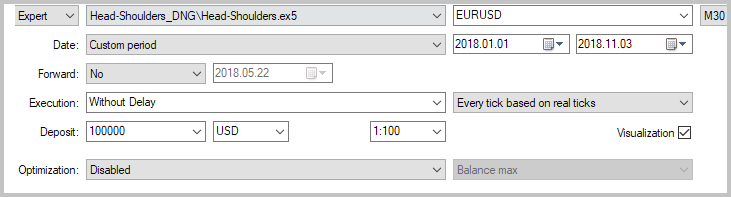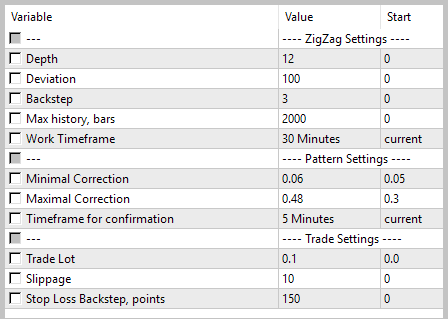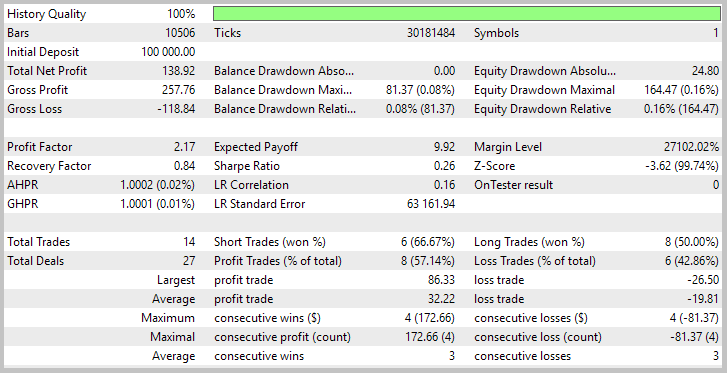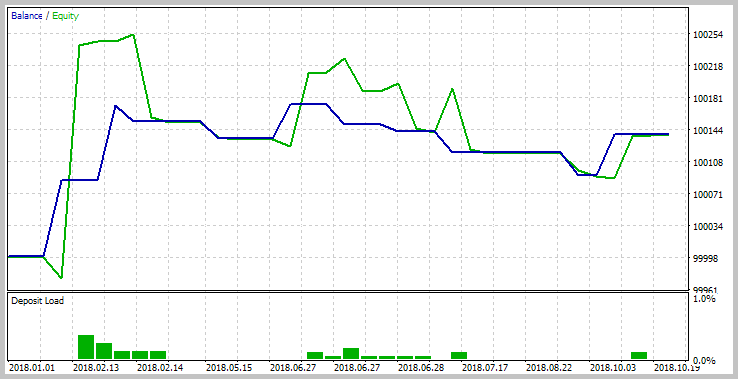### 4. 将两种形态合并为单一 EA

```int CPattern::Compare(const CPattern *node,const int mode=0) const
{
if(Type()>node.Type())
return -1;
else
if(Type()<node.Type())
return 1;
//---
if(s_StartTrend.TimeStartBar>node.StartTrend().TimeStartBar)
return -1;
else
if(s_StartTrend.TimeStartBar<node.StartTrend().TimeStartBar)
return 1;
//---
if(s_StartCorrection.TimeStartBar>node.StartCorrection().TimeStartBar)
return -1;
else
if(s_StartCorrection.TimeStartBar<node.StartCorrection().TimeStartBar)
return 1;
//---
if(s_EndCorrection.TimeStartBar>node.EndCorrection().TimeStartBar)
return -1;
else
if(s_EndCorrection.TimeStartBar<node.EndCorrection().TimeStartBar)
return 1;
//---
return 0;
}
```

```CPattern *NewClass(int type)
{
switch(type)
{
case 0:
return new CPattern();
break;
case 1:
return new CHS_Pattern();
break;
}
//---
return NULL;
}
```

```void OnTick()
{
//---
.........................
.........................
.........................
//---
for(int pat=0;pat<2;pat++)
{
Pattern=NewClass(pat);
if(CheckPointer(Pattern)==POINTER_INVALID)
return;
if(!Pattern.Create(ar_Objects.At(1),d_MinCorrection,d_MaxCorrection))
{
delete Pattern;
continue;
}
//---
datetime ss=start_search;
while(!IsStopped() && Pattern.Search(ss))
{
ss=fmax(ss,Pattern.EndTrendTime()+PeriodSeconds(e_TimeFrame));
bool found=false;
for(int i=2;i<ar_Objects.Total();i++)
{
CPattern *temp=ar_Objects.At(i);
if(Pattern.Compare(temp,0)==0)
{
found=true;
break;
}
}
if(found)
continue;
if(!CheckPattern(Pattern))
continue;
continue;
Pattern=NewClass(pat);
if(CheckPointer(Pattern)==POINTER_INVALID)
break;
if(!Pattern.Create(ar_Objects.At(1),d_MinCorrection,d_MaxCorrection))
{
delete Pattern;
break;
}
}
if(CheckPointer(Pattern)!=POINTER_INVALID)
delete Pattern;
}
//---
return;
}
```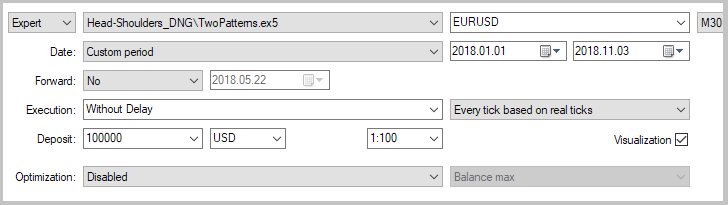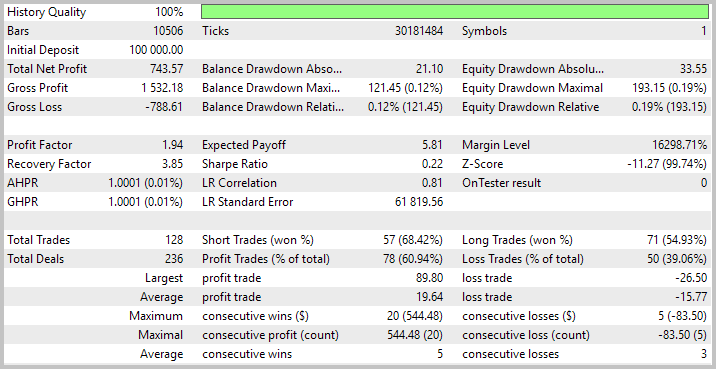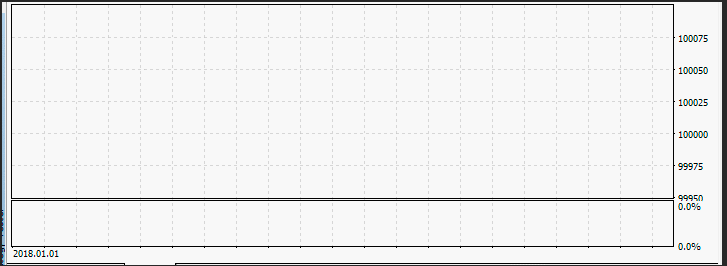### 5. 测试交易系统

EURUSD 743.57 1.94
EURJPY 125.13 1.47
GBPJPY
33.93 1.04
EURGBP -191.7
0.82
GBPUSD -371.05 0.60
USDJPY
-657.38 0.31

EURUSD 1020.28 1.78 350
EURJPY 532.54 1.52 400
GBPJPY 208.69 1.17 300
EURGBP 91.45 1.05 450
GBPUSD -315.87 0.55 100
USDJPY -453.08 0.33 100

### 6. 在亏损品种上分配交易信号

`input bool  ReverseTrade   =  true; //反向交易`

```bool CheckPattern(CPattern *pattern)
{
int signal=0;
double sl=-1, tp1=-1, tp2=-1;
if(!pattern.CheckSignal(signal,sl,tp1,tp2))
return false;
//---
double price=0;
double to_close=100;
double temp=0;
//---
```

```//---
switch(signal)
{
case 1:
CLimitTakeProfit::Clear();
{
to_close-=(fabs(tp1-tp2)>=_Point ? 50 : 100);
return false;
return false;
}
else
{
price=SymbolInfoDouble(_Symbol,SYMBOL_BID);
temp=tp1;
tp1=sl-i_SL*_Point;
sl=temp;
tp1=(price-tp1)/_Point;
tp2=(tp2-price)/_Point;
to_close-=((tp2-tp1)>=1 ? 50 : 100);
return false;
return false;
}
break;
case -1:
CLimitTakeProfit::Clear();
{
price=SymbolInfoDouble(_Symbol,SYMBOL_BID);
to_close-=(fabs(tp1-tp2)>=_Point ? 50 : 100);
return false;
return false;
}
else
{
temp=tp1;
tp1=sl+i_SL*_Point;
sl=temp;
tp1=(tp1-price)/_Point;
tp2=(price-tp2)/_Point;
to_close-=((tp2-tp1)>=1 ? 50 : 100);
return false;
return false;
}
break;
}
//---
return true;
}
```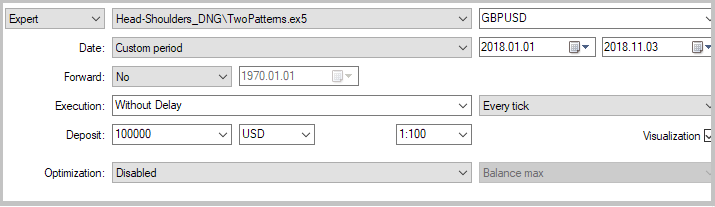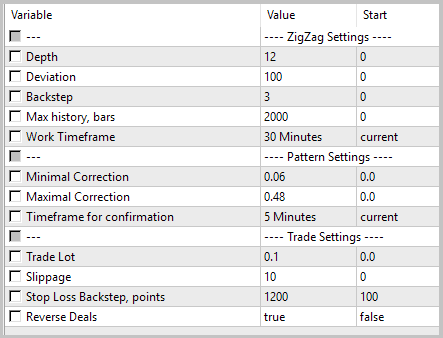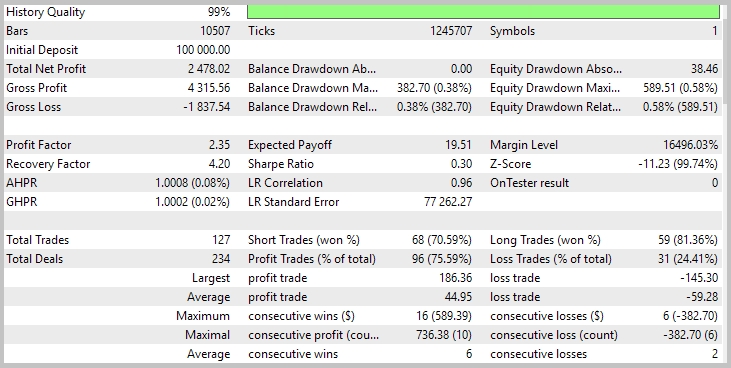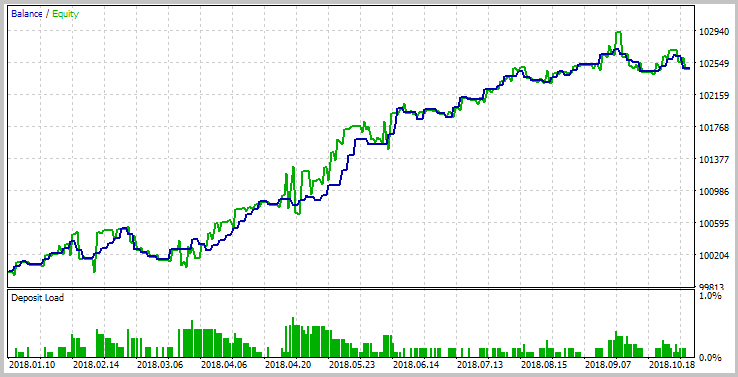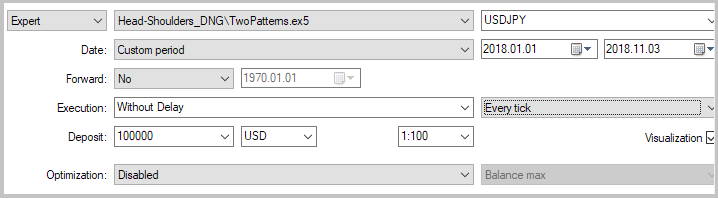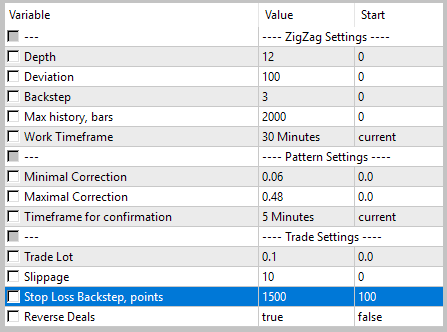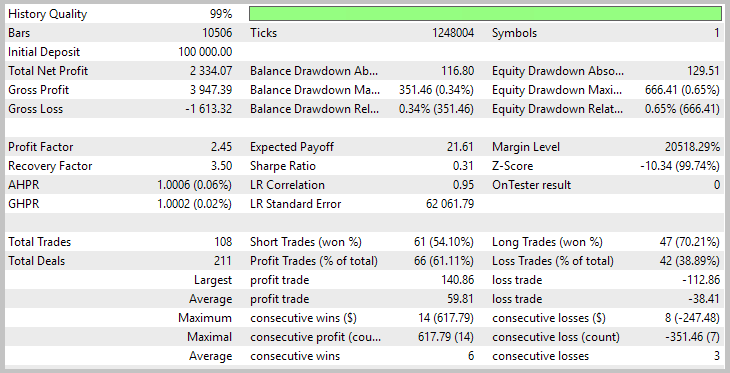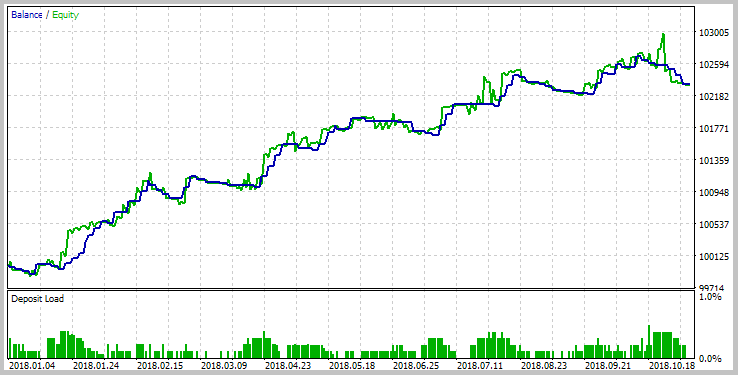### 本文中使用的程序

# 名称 类型 描述
1 ZigZag.mqh 类库 之字折线指标类
2 Trends.mqh 类库 趋势搜索类
3 Pattern.mqh 类库 处理双顶/双底形态的类
4 HS_Pattern 类库 处理头肩形态的类
5 LimitTakeProfit.mqh 类库 用限价订单替换止盈的类
8 TwoPatterns.mq5 智能交易系统 结合了两种形态的 EA

MQL5.zip (1352.35 KB)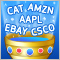反向交易: 减少最大回撤以及在其它市场上测试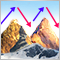逆转形态：测试双顶/双底形态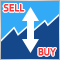逆转：正规化入场点并开发手动交易算法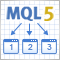自己动手开发多线程异步 MQL5 WebRequest